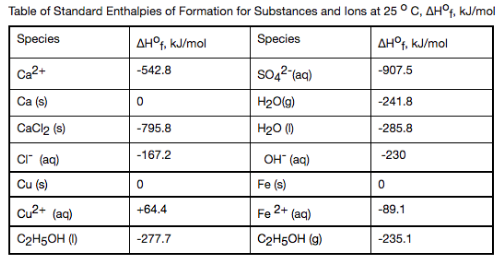# Problem: Calculate the enthalpy of reaction (in kJ) for 5.6 g of iron solid is reacted with aqueous Copper sulfate.

###### FREE Expert Solution
• To find the enthalpy of reaction, we need to find the enthalpy of formation of each product and reactant.

• This can be solved using the equation ΔHrxn = ΔHfprod - ΔHf react

• First step is to write the equation:###### Problem Details

Calculate the enthalpy of reaction (in kJ) for 5.6 g of iron solid is reacted with aqueous Copper sulfate.What scientific concept do you need to know in order to solve this problem?

Our tutors have indicated that to solve this problem you will need to apply the Enthalpy of Formation concept. You can view video lessons to learn Enthalpy of Formation. Or if you need more Enthalpy of Formation practice, you can also practice Enthalpy of Formation practice problems.

What is the difficulty of this problem?

Our tutors rated the difficulty ofCalculate the enthalpy of reaction (in kJ) for 5.6 g of iron...as high difficulty.

How long does this problem take to solve?

Our expert Chemistry tutor, Dasha took 7 minutes and 29 seconds to solve this problem. You can follow their steps in the video explanation above.

What professor is this problem relevant for?

Based on our data, we think this problem is relevant for Professor Ward & Paul's class at PITT.